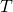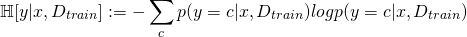Classification Probability in Deep Networks (Bayesian Deep Learning Part III)

In a previous post, I described Yarin Gal’s interpretation of dropout. This implementation allows us to generate an approximate distribution across the output of a deep neural network via Monte Carlo sampling, implemented through inference time dropout. I previously described how to use this distribution for uncertainty in regression, where the concepts of probability apply in a fairly straightforward way. In this post, I will describe how these distributions are used in classification problems.

While regression seeks to map an input to a numerical output, classification seeks to map an input to a categorical output (cat, dog, bird, etc).  These categorical outputs do not lend themselves to expected values and covariances in the same way a regression problem would, so we have to determine another way to characterize the distribution.

First, let’s think about what our Monte Carlo integration is giving us. Every inference gives us a distribution across our categorical outputs, and all of these distributions are combined to one output distribution. An example distribution may look like this:This distribution has the highest probability for cat, with dog and squirrel running close behind. This distribution is neither Gaussian nor unimodal, which means that our mean and covariance statistics will not function in this case (though if the categorical outputs are contiguous, such statistics may be justifiable). So our guiding question is: how do we properly describe our confidence in this distribution’s output?

Gal suggests three measures for determining the spread of the distribution. The first, variation ratio, is calculated as, whereis the number of samples, andis the number of times the network’s highest value is the same as the mode of highest values across all forward passes. By counting the number of times the mode answer is selected, it approximates.

A second measure, predictive entropy, averages the distribution across all of our Monte Carlo samples, then runs them through a softmax operator, giving a biased estimator of the form:Where ouris the softmaxed average output for each class,.

Closely related is the mutual information metric. This metric provides a measure of how consistent the model is across multiple MC passes (Gal uses the term “model’s confidence”), while variation ratio and predictive entropy measure how likely the output is to be correct (“uncertainty in the output”). The equation for mutual information is:Conclusion

These three methods for determining the certainty in classification conclude the high level, and possibly slightly incorrect, tour of the method described in Yarin Gal’s thesis. His proposed approach allows us to develop the critically necessary notion of uncertainty while leveraging the power of deep networks. The downside is, of course, that these approximations require many forward passes. This may not be an issue where power and time are comparatively cheap, such as medical diagnosis. However, with power-limited hardware or real time requirements (cellphones and robots), we may need to reframe networks yet again to get a good answer.

But perhaps by the time we figure that out, Moore’s law would have made it all a moot point.

If you’re interested in this subject, I would definitely recommend reading Yarin Gal’s thesis. One of his former labmates, Alex Kendall, also wrote a quite informative blog post arguing for this line of research. Gal has, of course, published many other papers on the subject. If you’re intimately familiar with Gaussian Processes, you may be more comfortable with these, but I found his thesis to be the most digestable of all I read.

Posted on Categories Uncategorized

This site uses Akismet to reduce spam. Learn how your comment data is processed.